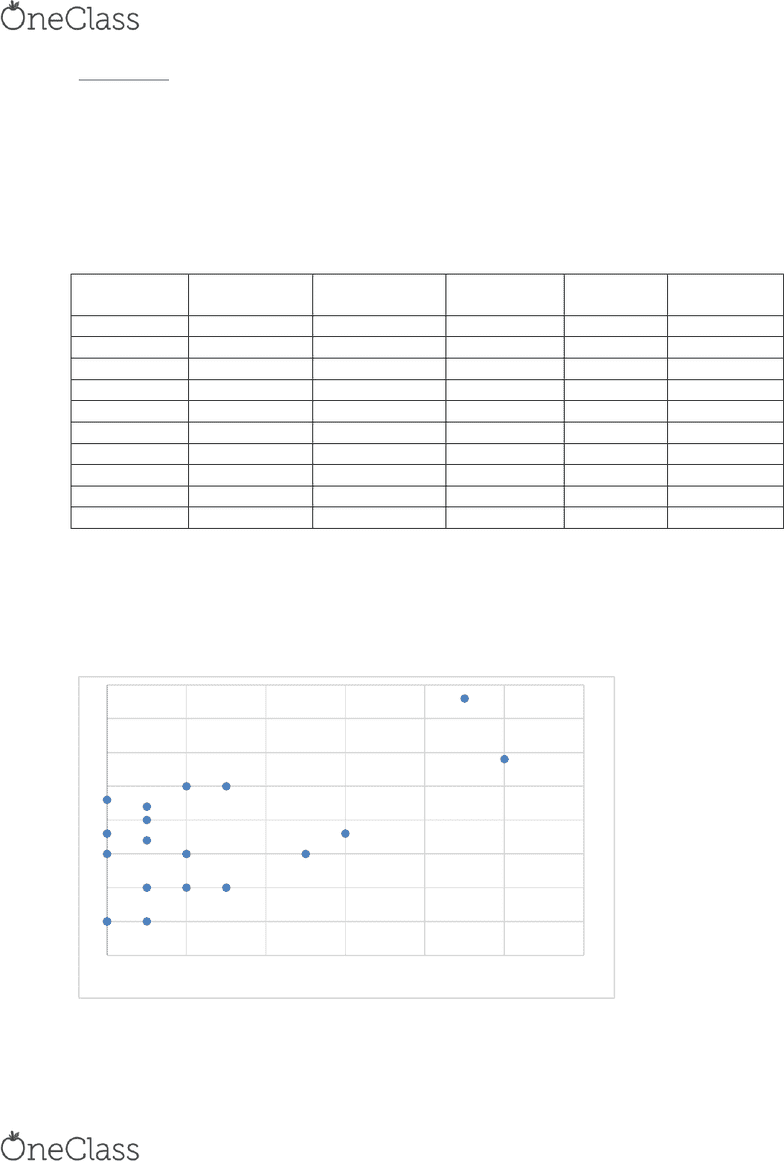Class Notes (1,100,000)
CA (640,000)
UTSC (30,000)
HLTB21H3 (200)
Lecture 7

# HLTB21H3 Lecture Notes - Lecture 7: Simple Linear Regression, Confidence Interval, Null Hypothesis

Department
Health Studies
Course Code
HLTB21H3
Professor
Caroline Barakat
Lecture
7

This preview shows pages 1-3. to view the full 32 pages of the document.Application 1
A study by Triller et al. (A-14) examined the length of time required for home health-care nurses to
repackage a patient’s medications into various medication organizers (i.e., pill boxes). For the 19 patients
in the study, researchers recorded the time required for repackaging of medications. They also recorded
the number of problems encountered in the repackaging sessions.
Patient No.
No. of
Problems
Repackaging
Time (min)
Patient No.
No. of
Problems
Repackaging
Time (min)
1
9
38
11
1
10
2
2
25
12
2
15
3
0
5
13
1
17
4
6
18
14
0
18
5
5
15
15
0
23
6
3
25
16
10
29
7
3
10
17
0
5
8
1
5
18
1
22
9
2
10
19
1
20
10
0
15
Dependent Variable: Repackaging Time
Independent Variable: Number of Problems
a. scatter diagram
0
5
10
15
20
25
30
35
40
0 2 4 6 8 10 12
Repackacking Time
find more resources at oneclass.com
find more resources at oneclass.com

Only pages 1-3 are available for preview. Some parts have been intentionally blurred.b. Assuming a linear relationship, find the simple linear regression equation.
(Excel Summary Output of the Regression Analysis)
Multiple R
0.616798
R Square
0.38044
Square
0.343995
Standard Error
7.080977
Observations
19
ANOVA
df
SS
MS
F
Significance
F
Regression
1
523.4054
523.4054
10.43883
0.00491
Residual
17
852.3841
50.14024
Total
18
1375.789
Coefficients
Standard
Error
t Stat
P-value
Lower 95%
Upper
95%
Lower
95.0%
Upper
95.0%
Intercept
12.64145
2.132547
5.927867
1.65E-05
8.142173
17.14073
8.142173
17.14073
X Variable 1
1.804519
0.558516
3.230918
0.00491
0.626153
2.982884
0.626153
2.982884
The regression equation is

c. Interpret the meaning of the Y intercept bo and the slope b1 in this problem.
bo= the portion of repackaging time not explained by number of problems encountered
find more resources at oneclass.com
find more resources at oneclass.com

Only pages 1-3 are available for preview. Some parts have been intentionally blurred.b1= for each additional one problem encountered there is an average increase of 1.8 min spent repackaging by nurse.
d. Use the regression equation developed to predict the time spent for a nurse who has 10
problems.

e. Determine the coefficient of correlation r. Interpret its meaning in this application.
r=.617 tells you that there is a moderately strong association (linear) between number of
problems encountered and repackaging time.
f. Determine the coefficient of determination r2 and interpret its meaning in the context of this
application.
r2=0.38 38% of the variation in the repackaging time is explained by the variation in the
number of problems encountered by a nurse.
g. Determine the standard error of the estimate and interpret its meaning in the context of this
application.
SYX= 7.081 is the standard deviation of the observed repackaging times spent (by nurses)
around the line of regression. 95% of the observed times spent will fall within 2 x 7.081of
the least squares line (regression line).
h. At the 0.01 level of significance, is there evidence of a linear relationship between the time
spent and the number of topics researched?
Perform the t test for the significance of slope between number of problems encountered
and time spent by nurses repackaging. (2 tail. test)
H0:=0 there is not a linear relationship between repackaging time and number of
problems encountered.
H1:≠0 there is a linear relationship between repackaging time and number of problems
encountered.

 

Critical value for the test

Conclusion: At  There is a linear relationship between number of
problems encountered and repackaging time.
find more resources at oneclass.com
find more resources at oneclass.com